Question

# Use the Gauss-Jordan method to solve the following system of equations. 3x + 4y - 2z...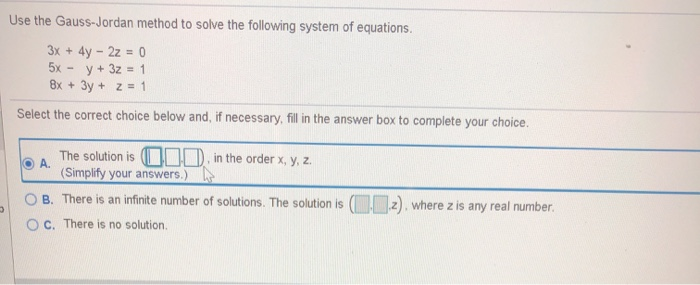Use the Gauss-Jordan method to solve the following system of equations. 3x + 4y - 2z = 0 5x y + 3z = 1 8x + 3y + z = 1 Select the correct choice below and, if necessary, fill in the answer box to complete your choice. A. The solution is 000 in the order x, y, z. (Simplify your answers.) B. There is an infinite number of solutions. The solution is (Q10.2), where z is any real number OC. There is no solution 3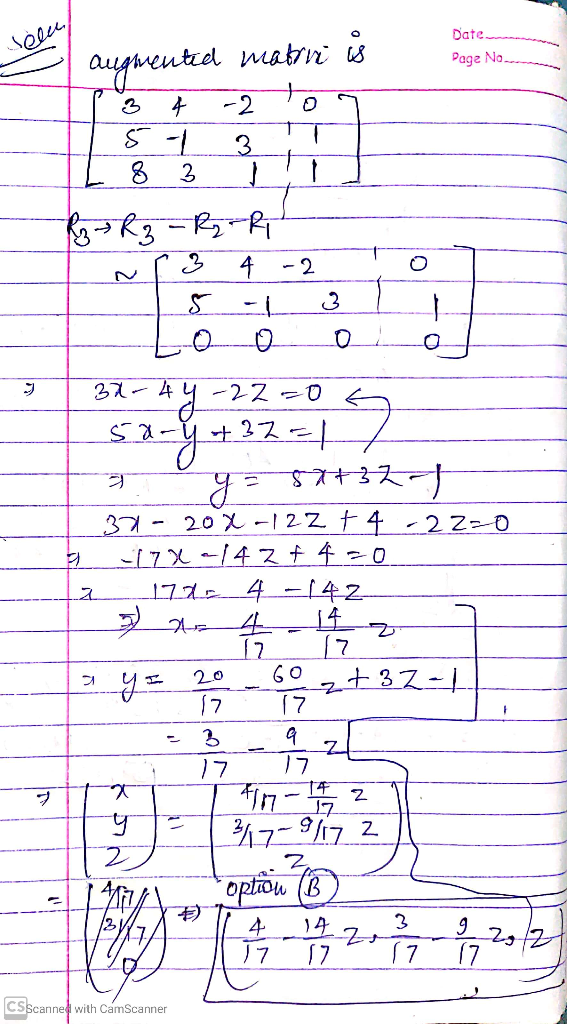Please thumbs up the solution. Thanks

#### Earn Coins

Coins can be redeemed for fabulous gifts.

Similar Homework Help Questions
• ### Use the​ Gauss-Jordan method to solve the following system of equations. 5x+4y-3z+0 2x-y+5z=1 7x+3y+2z=1 Multiple Choice...

Use the​ Gauss-Jordan method to solve the following system of equations. 5x+4y-3z+0 2x-y+5z=1 7x+3y+2z=1 Multiple Choice A.The solution is B.There is an infinite number of solutions. The solution is C. There is no solution.

• ### Solve the following system of equations using Gaussian or Gauss-Jordan elimination X- 3y + 3z =...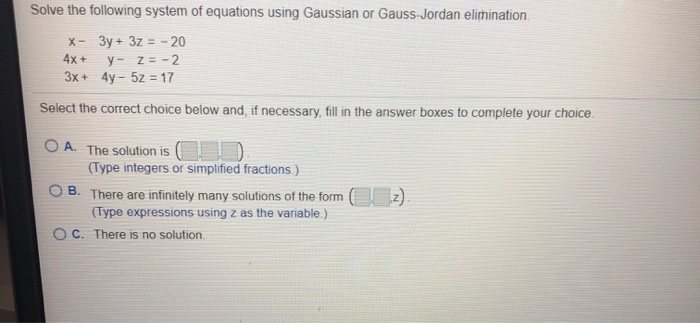Solve the following system of equations using Gaussian or Gauss-Jordan elimination X- 3y + 3z = -20 4x + y - Z= -2 3x + 4y - 5z = 17 Select the correct choice below and, if necessary, fill in the answer boxes to complete your choice. O A. The solution is C (Type integers or simplified fractions) OB. There are infinitely many solutions of the form ez) (Type expressions using z as the variable.) C. There is no solution

• ### Use the Gauss Jordan method to solve the following system of equations. 4x - 9y = 5 8x - 18y = 1

Use the Gauss Jordan method to solve the following system of equations. 4x - 9y = 5 8x - 18y = 1 Select the correct choice below and, if necessary, fill in the answer box to complete your choice. A. The solution is _______ B. There are infinitely many solutions. The solution is ( _______ , y) where y is any real number. C. There is no solution.

• ### Solve the following system of equations using Gaussian or Gauss-Jordan elimination. X- 2y + 4z =...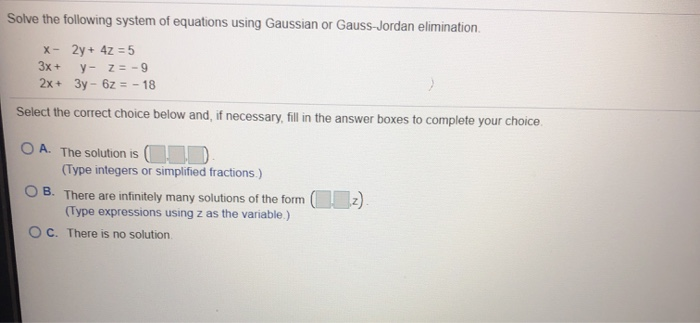Solve the following system of equations using Gaussian or Gauss-Jordan elimination. X- 2y + 4z = 5 3x + y- Z = -9 2x + 3y - 6z = - 18 Select the correct choice below and, if necessary, fill in the answer boxes to complete your choice O A. The solution is c. (Type integers or simplified fractions.) OB. There are infinitely many solutions of the form (2) (Type expressions using z as the variable.) OC. There is no...

• ### Use the Gauss-Jordan method to solve the following system of equations. 7x - 2y = 5 28x - 8y = 20

Use the Gauss-Jordan method to solve the following system of equations. 7x - 2y = 5 28x - 8y = 20 Select the correct choice below and, if necessary, fill in the answer box to complete your choice. A. The solution is_______ B. There are infinitely many solutions. The solution is (_______ ,y), where y is any real number.C. There is no solution.

• ### Solve the system of linear equations, using the Gauss-Jordan elimination method. (If there is no ...

Solve the system of linear equations, using the Gauss-Jordan elimination method. (If there is no solution, enter NO SOLUTION. If there are infinitely many solutions, express your answer in terms of the parameters t and/or s.) x − 2y + 3z = 3 2x + 3y − z = 0 x + 2y − 3z = −7 (x, y, z) = ( )

• ### Solve the system of equations. X+y-z=1 3x-y+z=7 x- 4y + 2z = -18 Select the correct...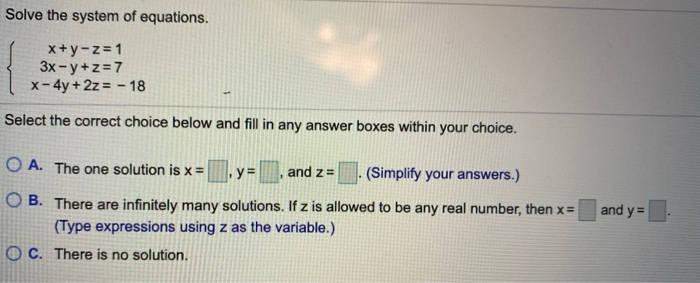Solve the system of equations. X+y-z=1 3x-y+z=7 x- 4y + 2z = -18 Select the correct choice below and fill in any answer boxes within your choice. O A. The one solution is x = 0, y = , and z = (Simplify your answers.) O B. There are infinitely many solutions. If z is allowed to be any real number, then x= (Type expressions using z as the variable.) O C. There is no solution. and y=

• ### Use the Gauss-Jordan method to solve the equation: 3x-5y-2z=-9 -4x+3y+z=11 8x-5y+4z=6

Use the Gauss-Jordan method to solve the equation:3x-5y-2z=-9-4x+3y+z=118x-5y+4z=6

• ### 1. Solve the following system of equations using Gauss-Jordan elimination. 3x - 2y +4z=3 2x +2y-2z=4...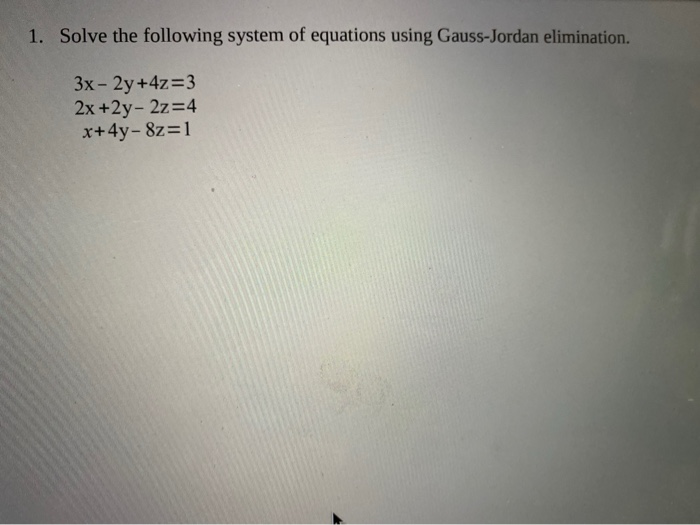1. Solve the following system of equations using Gauss-Jordan elimination. 3x - 2y +4z=3 2x +2y-2z=4 x+4y- &z=1

• ### Solve the system by using Gaussian elimination or Gauss-Jordan elimination. -- 5x+12y + 5z = -55...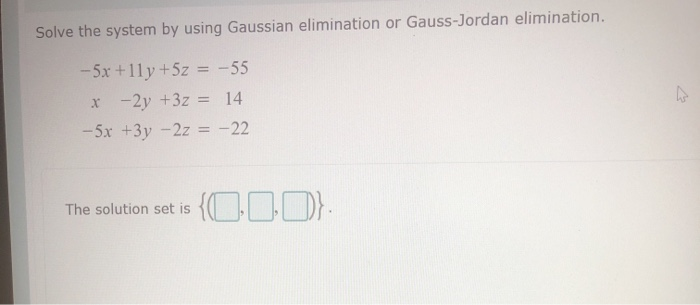Solve the system by using Gaussian elimination or Gauss-Jordan elimination. -- 5x+12y + 5z = -55 x-2y +3z = 14 -5x +3y – 2z = -22 The solution set is {000}: